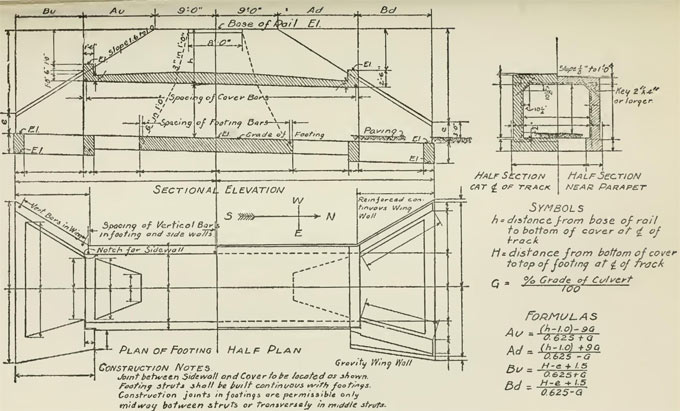# Various types of notations used in the construction of concrete culvert designThe following notations are used in the drawing of concrete culvert design.

With notation, it is possible to make clear communication among various project stakeholders. Notation helps in avoiding mistakes in any construction project.

A? = The valid contact area of a footing, measuring unit is in square metres
A = A detailing dimension for culverts that contains skewed ends, measuring unit is in mm
B = Depth of the bottom slab of a box culvert, measuring unit is in mm
B = Gapping among adjoining bars with reference to detailing tables, measuring unit is in mm
C = A coefficient that is applied in finding out the quantities of reinforcing bar.
CANBAS = Canadian Bridge Analysis System
c? = the valid cohesion among the base of the footing and the soil at the ULS, with reference to CHBDC. kPa
CHBDC = Canadian Highway Bridge Design Code, 2000 Edition
CGSB = Canadian General Standards Board
F = Width of footing for open footing culverts, measuring unit is in mm
F1 = A reinforcing bar spacing factor, measuring unit is in mm-1
HULS = maximum factored horizontal reaction at the level of the base of the footing at the ULS, kN
Lc = Culvert length that is calculated the longitudinal axis, measuring unit is in m
OCPA = Ontario Concrete Pipe Association

OMBAS = Ontario Modular Bridge Analysis System
OPSS = Ontario Provincial Standard Specifications
S = Culvert distance that is calculated perpendicular to the longitudinal axis of the culvert, measuring unit is in mm
SLS = Serviceability limit states, in accordance with CHBDC

T = Depth of top slab of culvert, mm tan f? effective friction coefficient for concrete cast against soil.
ULS = Ultimate limit states, with reference to CHBDC
V = Unfactored vertical reaction because of the dead load of cast-in-place concrete and soil fill, at the level of the base of the footing, kN
VSLS = Maximum vertical reaction at the level of the base of the footing at SLS, kN

VULS = Maximum factored vertical reaction at the level of the base of the footing at ULS, kN
W = Depth of wall of culvert, measuring unit is in mm
G = The extreme angle among the normal to the longitudinal axis and the end of the same culvert, degrees
f? = The valid angle of internal friction, with reference to CHBDC, measuring unit is in degrees
? = Skew angle of culvert, degrees